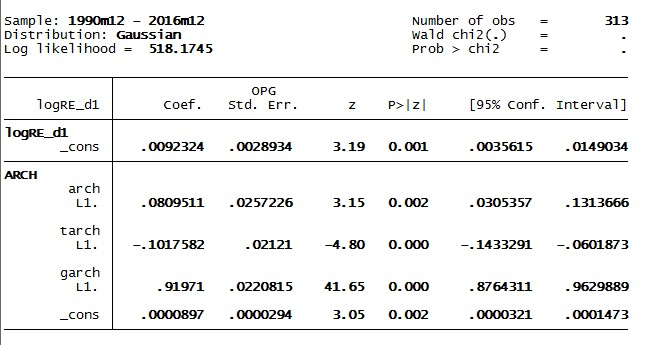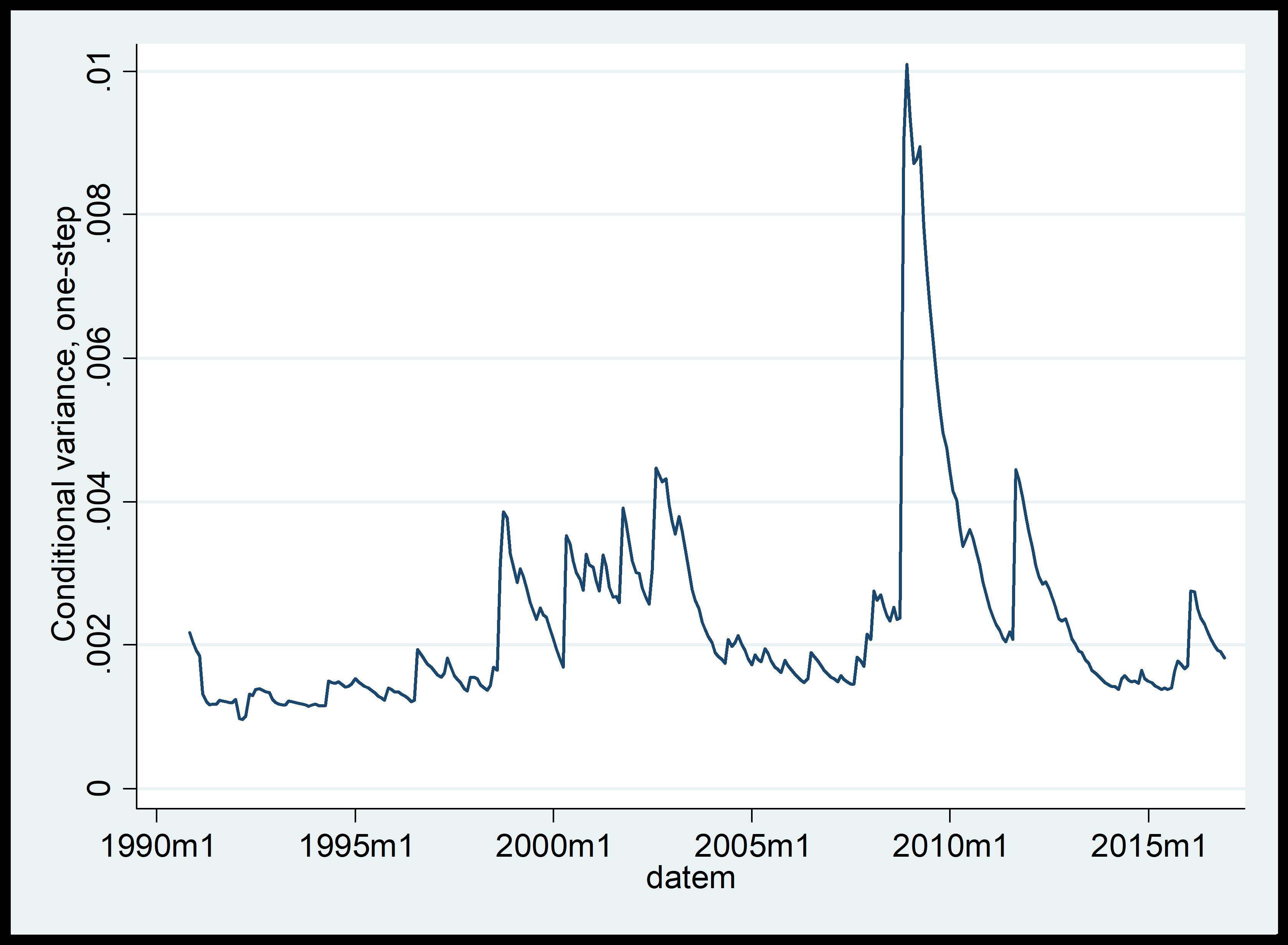# Time series using GARCH model in STATA

The previous article showed time series using autoregressive conditional hetroskedasticity (ARCH) model in STATA. The present article shows extensions of ARCH, i.e. GARCH model in STATA. Like ARCH model, ARCH extensions like Generalised ARCH (GARCH) model also need squared residuals as determinants of the equation’s variance.

## Generalized ARCH (GARCH) model

In GARCH model, the conditional variance of error term at time ‘t’ depends on the following:

• Squared error term in the previous time period (ARCH1)
• Its conditional variance in the previous time period.

Follow this command for using GARCH model:

`arch  logRE_d1, arch(1) garch(1)`

The interpretation of the syntax is as follows.

In the ARCH regression model, ‘logRE_d1’ is a dependent variable with no independent variables other than a constant. ‘arch(1)’ command adds a single lagged value of et to the modelled variance in STATA. ‘garch(1)’ command adds a single lag of the variance, ht, to the modelled variance.

## Results of GARCH model

The figure below shows the results of GARCH model. The estimate of a lagged value of error term is 0.491 whereas the coefficient estimate is 0.238. The p values are all significant and log likelihood is also highest in all the iterations.Figure 1: Results of GARCH model in STATA

Like ARCH, generate variances for GARCH model using the same command:

`predict GTgarch, variance`

Here ‘GTgarch’ is the name for predicted series of variances. The results will not appear in ‘Result’ window, but in ‘data editor’ window of STATA. To examine the movement of GTgarch generates a time plot using this command:

STATA Command: tsline GTgarch

The figure below shows the results.Figure 2: Time plots of predicted variances under GARCH

The above graph shows that there is a lot more volatility towards the entire span of the sample considering the lagged values of variances.

## Threshold GARCH (TGARCH) model

The threshold GARCH model or TGARCH is another generalization of the GARCH model where positive and negative news are treated asymmetrically. This adds another option to the arch ‘Stock_RE_d1 regression model in STATA. Use this command for TGARCH model in STATA:

`arch  logRE_d1, arch(1) garch(1) tarch(1)`

The figure below shows the results. The estimate of the lagged value of error term is 0.08 and the coefficient on the lagged variance is 0.919. The p values are all significant and log likelihood is also highest in all the iterations.Figure 3: TGARCH results in STATA

Further to generate variances for TGARCH use this command:

`predict HTgarch, variance`

Here ‘HTgarch’ is the name for the predicted series of variances. The results do not appear in ‘Result’ window, but in ‘data editor’ window of STATA. To examine the movement of the variance of HTgarch, generate a time plot using this command:

`tsline HTgarch`Figure 4: Time plots of predicted variances under TGARCH

After analyzing ARIMA, VAR, VEC, ARCH and some extensions of the ARCH models, the next set of articles will focus upon some common diagnostics tests applied to time series analysis. These tests will pertain to normality, heteroskedasticity, autocorrelation, multicollinearity and stability.

### Divya Dhuria

Research analyst at Project Guru
DIvya has a keen interest in policy making and wealth management. She is a Master in Economics from Gokhale Institute of Politics and Economics. She has been trained in the econometric techniques to assess different possible economic relationships. She hascontributed to the working paper on National Rural Health Mission at Institute of economic growth, Delhi. Along with academical growth, she likes to explore and visit different places in her spare time.

#### Latest posts by Divya Dhuria (see all)We are looking for candidates who have completed their master's degree or Ph.D. Click here to know more about our vacancies.